# Solids Liquids Gases Chapter 3 Solids Liquids Gases

• Slides: 15Solids, Liquids, & Gases Chapter 3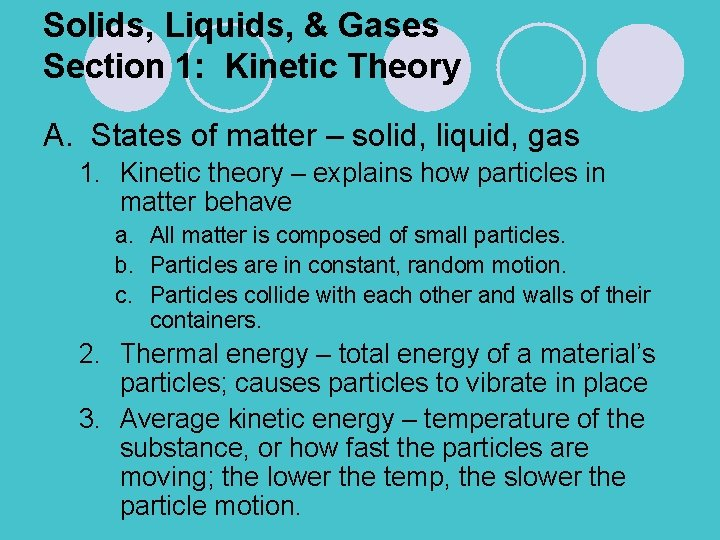Solids, Liquids, & Gases Section 1: Kinetic Theory A. States of matter – solid, liquid, gas 1. Kinetic theory – explains how particles in matter behave a. All matter is composed of small particles. b. Particles are in constant, random motion. c. Particles collide with each other and walls of their containers. 2. Thermal energy – total energy of a material’s particles; causes particles to vibrate in place 3. Average kinetic energy – temperature of the substance, or how fast the particles are moving; the lower the temp, the slower the particle motion.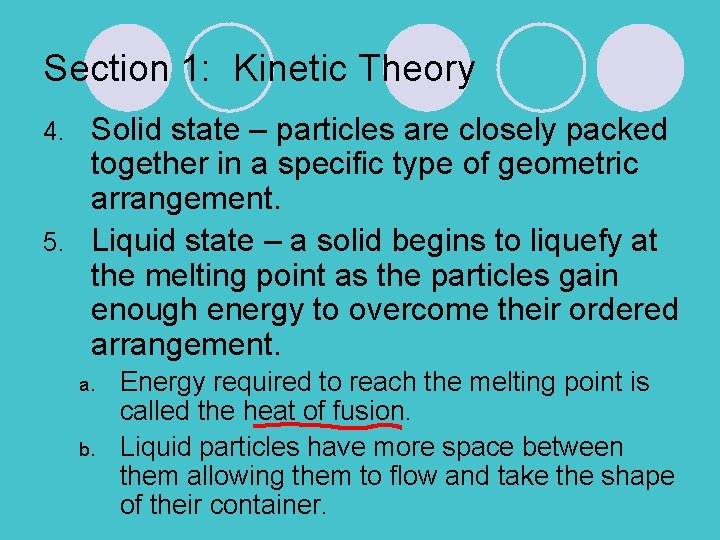Section 1: Kinetic Theory Solid state – particles are closely packed together in a specific type of geometric arrangement. 5. Liquid state – a solid begins to liquefy at the melting point as the particles gain enough energy to overcome their ordered arrangement. 4. a. b. Energy required to reach the melting point is called the heat of fusion. Liquid particles have more space between them allowing them to flow and take the shape of their container.Section 1: Kinetic Theory 6. Gaseous state – a liquid’s particles have enough energy to escape the attractive forces of the other particles in the liquid. a. Heat of vaporization is the energy required for a liquid to change to a gas. b. At the boiling point, the pressure of the liquid’s vapor is equal to the pressure of the atmosphere, and that liquid becomes a gas. c. Gas particles spread evenly throughout their container in the process of diffusion.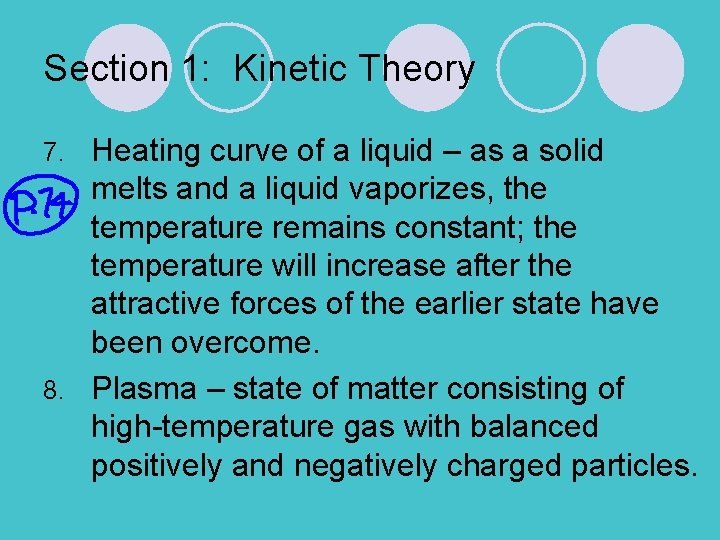Section 1: Kinetic Theory Heating curve of a liquid – as a solid melts and a liquid vaporizes, the temperature remains constant; the temperature will increase after the attractive forces of the earlier state have been overcome. 8. Plasma – state of matter consisting of high-temperature gas with balanced positively and negatively charged particles. 7.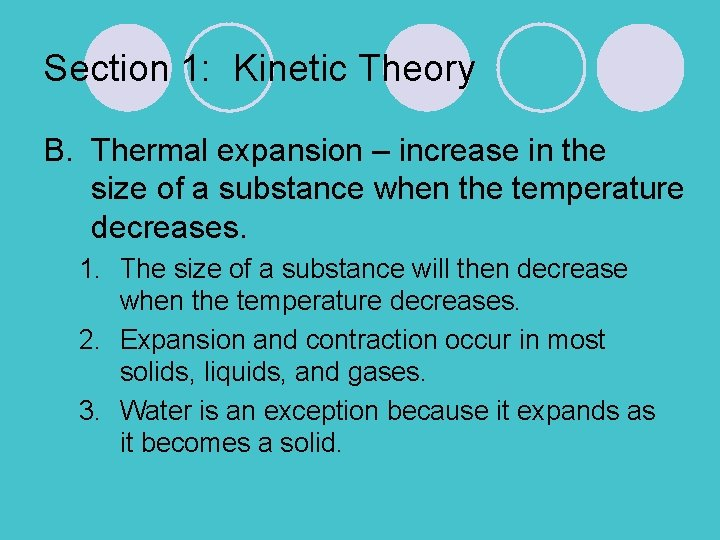Section 1: Kinetic Theory B. Thermal expansion – increase in the size of a substance when the temperature decreases. 1. The size of a substance will then decrease when the temperature decreases. 2. Expansion and contraction occur in most solids, liquids, and gases. 3. Water is an exception because it expands as it becomes a solid.Section 1: Kinetic Theory C. Some substances do not react as expected when changing states. 1. Amorphous solids – lack the tightly ordered structure found in crystals a. Do not have definite temperature at which they change from solid to liquid b. Glass, plastic 2. Liquid crystals do not lose their ordered arrangement completely upon melting; used in liquid crystal displays in watches, clocks, calculators, and some notebook computers.Discussion Question #1 How are temperature and kinetic energy related? Temperature and kinetic energy are related because temperature is the average kinetic energy of a substance. As the temperature increases, the particles gain more kinetic energy or move faster.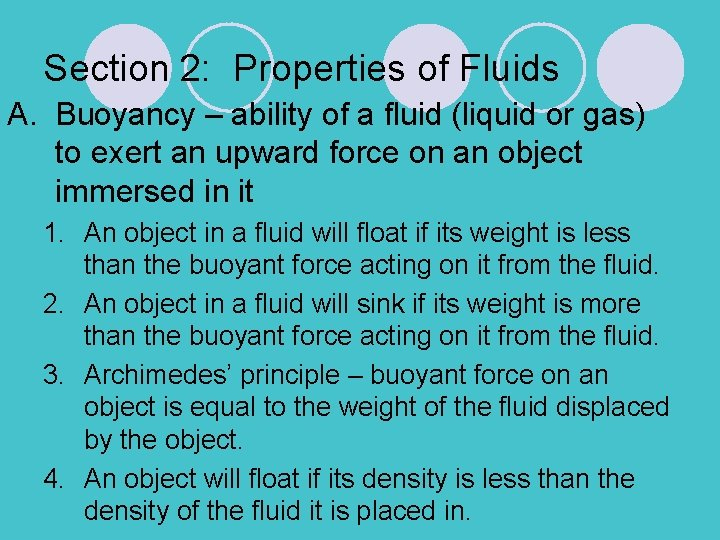Section 2: Properties of Fluids A. Buoyancy – ability of a fluid (liquid or gas) to exert an upward force on an object immersed in it 1. An object in a fluid will float if its weight is less than the buoyant force acting on it from the fluid. 2. An object in a fluid will sink if its weight is more than the buoyant force acting on it from the fluid. 3. Archimedes’ principle – buoyant force on an object is equal to the weight of the fluid displaced by the object. 4. An object will float if its density is less than the density of the fluid it is placed in.Section 2: Properties of Fluid B. Pascal’s principle – pressure applied to a fluid is transmitted throughout the fluid 1. Pressure is force exerted per unit area. 2. Hydraulic machines use this principle to lift heavy loads. C. Bernoulli’s principle – as the velocity of a fluid increases, the pressure exerted by the fluid decreases; airplanes use this principle to fly.Section 2: Properties of Fluids D. Viscosity – a liquid’s resistance to flow 1. 2. Molecular structure determines a fluid’s viscosity. Increased temperature will lower viscosity.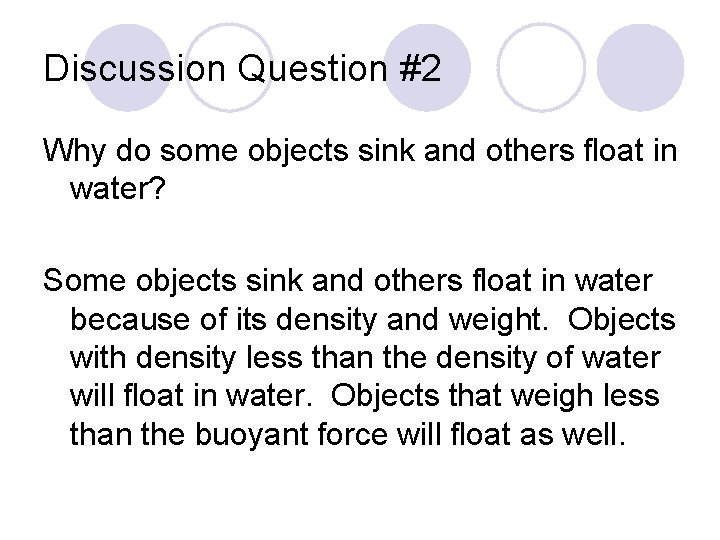Discussion Question #2 Why do some objects sink and others float in water? Some objects sink and others float in water because of its density and weight. Objects with density less than the density of water will float in water. Objects that weigh less than the buoyant force will float as well.Section 3: Behavior of Gases A. Pressure is measured in units called pascals (Pa). 1. 2. B. Collisions of particles in air result in atmospheric pressure. Moving particles colliding with the inside walls of a container result in gas pressure. Boyle’s Law – relates pressure and volume 1. 2. 3. Volume decreases as pressure increases Pressure decreases as volume increases Pressure multiplied by volume is always equal to a constant if the temperature is constant.Section 3: Behavior of Gases C. Charles’s Law – relates volume and temperature 1. At a constant pressure, volume increases as temperature increases 2. At a constant pressure, volume decreases as temperature decreases D. Gay-Lussac’s Law – relates pressure and temperature; at a constant volume, as a temperature increases, pressure increases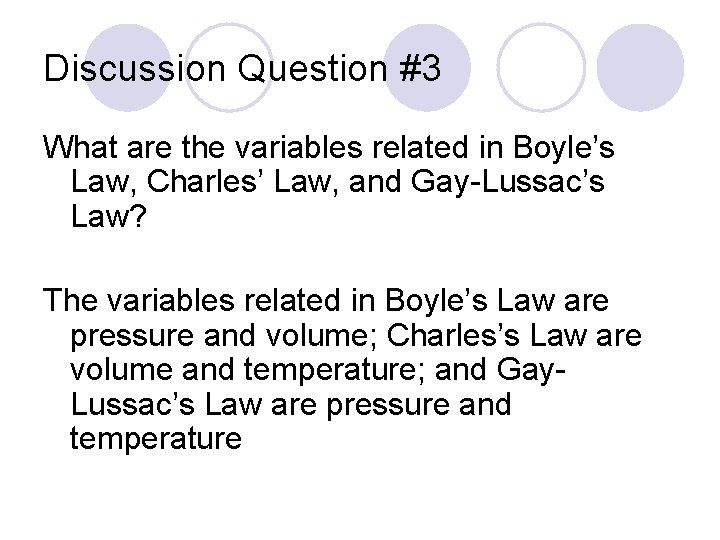Discussion Question #3 What are the variables related in Boyle’s Law, Charles’ Law, and Gay-Lussac’s Law? The variables related in Boyle’s Law are pressure and volume; Charles’s Law are volume and temperature; and Gay. Lussac’s Law are pressure and temperature